## An electron is constrained to the central perpendicular axis of a ring of charge of radius 2.2 m and charge 0.021 mC. Suppose the electron i

Question

An electron is constrained to the central perpendicular axis of a ring of charge of radius 2.2 m and charge 0.021 mC. Suppose the electron is released from rest a distance 0.050 m from the ring center. It then oscillates through the ring center. Calculate its period. (The electron is always much closer to the ring center than a radius.)

in progress 0
6 months 2021-08-05T22:07:28+00:00 1 Answers 8 views 0

## Answers ( )

T = 1.12 10⁻⁷ s

Explanation:

This exercise must be solved in parts. Let’s start looking for the electric field in the axis of the ring.

All the charge dq is at a distance r

dE = k dq / r²

Due to the symmetry of the ring, the field perpendicular to the axis is canceled, leaving only the field in the direction of the axis, if we use trigonometry

cos θ =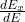dEₓ = dE cos θ

cos θ = x / r

substituting

dEₓ =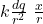DEₓ = k dq x / r³

let’s use the Pythagorean theorem to find the distance r

r² = x² + a²

where a is the radius of the ring

we substitute

dEₓ =we integrate

∫ dEₓ =k \frac{x}{(x^2 + a^2 ) ^{3/2} }  ∫ dq

Eₓ =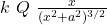In the exercise indicate that the electron is very central to the center of the ring

x << a

Eₓ =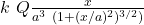if we expand in a series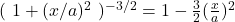we keep the first term if x<<a

Eₓ =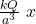the force is

F = q E

F =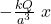this is a restoring force proportional to the displacement so the movement is simple harmonic,

F = m a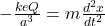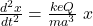the solution is of type

x = A cos (wt + Ф)

with angular velocity

w² =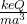angular velocity and period are related

w = 2π/ T

we substitute

4π² / T² = \frac{keQ}{m a^3}

T = 2π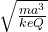let’s calculate

T = 2π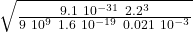T = 2π pi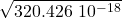T = 2π  17.9 10⁻⁹ s

T = 1.12 10⁻⁷ s